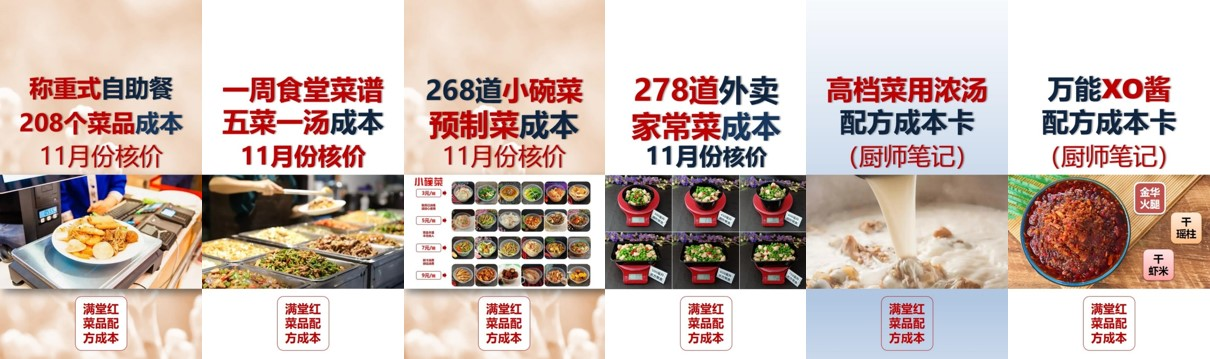做熟的菜，常用调料（油、味精、鸡精、综合谷氨酸钠、胡椒粉等）的含量是多少？固形物占比？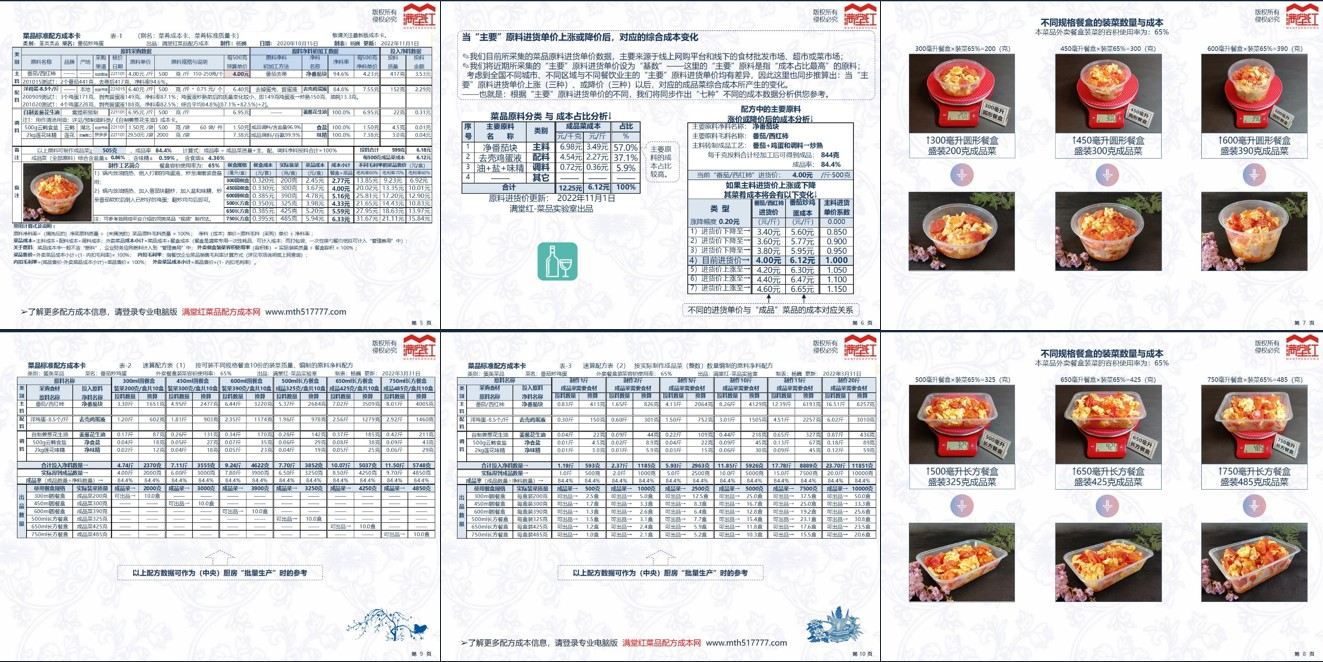（1）小碗菜菜谱↓    （2）外卖菜菜谱↓   （3）称重式自助餐              （4）天天不重样的五菜一汤食堂每周菜谱

家常菜菜谱↓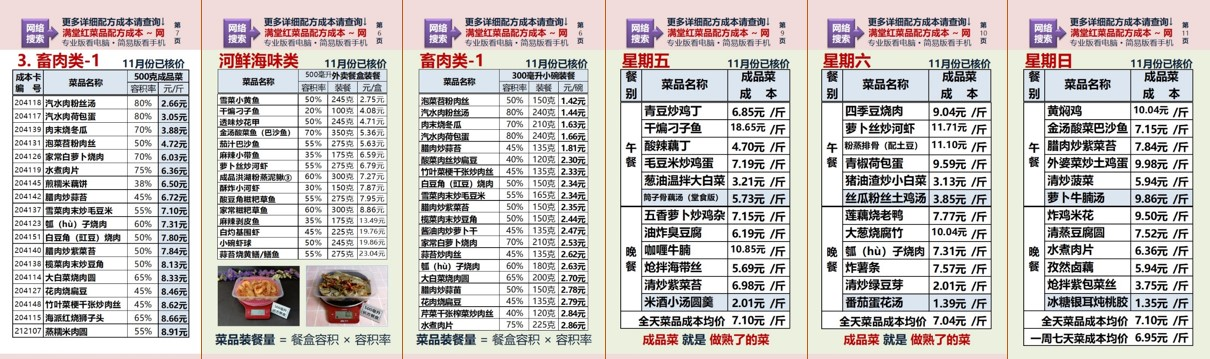➤做餐饮、从菜品成本核算开始！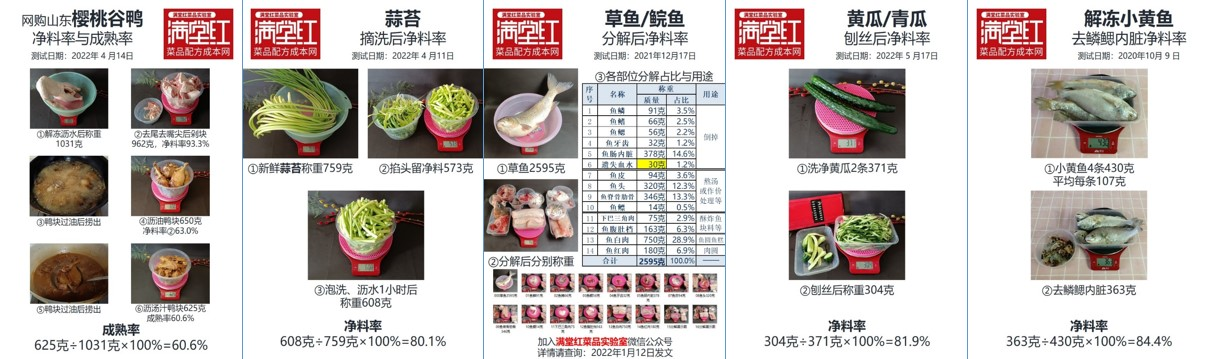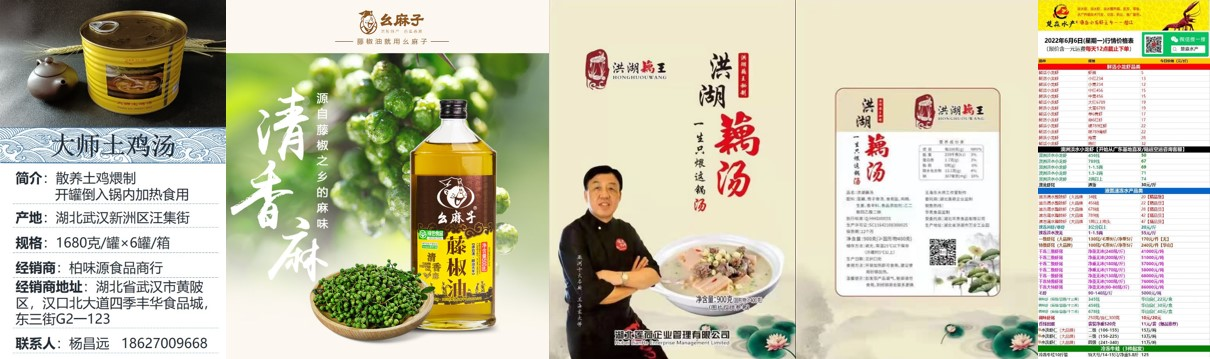：也就是无论多大的餐盒，装这道菜只需装满20%（克）即可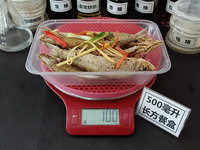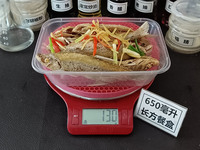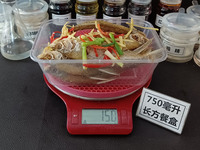用500毫升餐盒装餐 =500×20%=装餐100克 用650毫升餐盒装餐 =650×20%=装餐130克 用750毫升餐盒装餐 =750×20%=装餐150克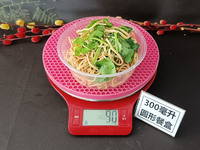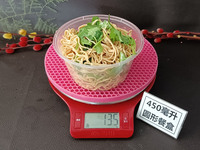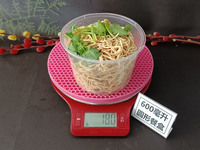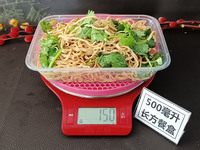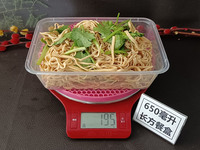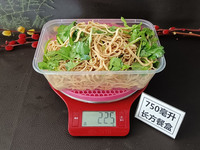用300毫升餐盒装餐=300×30%=装餐90克 用450毫升餐盒装餐=450×30%=装餐135克 用600毫升餐盒装餐=600×30%=装餐180克 用500毫升餐盒装餐=500×30%=装餐150克 用650毫升餐盒装餐=650×30%=装餐195克 用750毫升餐盒装餐=750×30%=装餐225克用300毫升餐盒装餐=300×40%=装餐120克 用450毫升餐盒装餐=450×40%=装餐180克 用600毫升餐盒装餐=600×40%=装餐240克 用500毫升餐盒装餐=500×40%=装餐200克 用650毫升餐盒装餐=650×40%=装餐260克 用750毫升餐盒装餐=750×40%=装餐300克